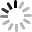# SVG D3.js - 時間格式 ( Time Formatting )• d3.time.format(specifier)
• format(date)
• format.parse(string)
• d3.time.format.multi(formats)
• d3.time.format.utc(specifier)
• d3.time.format.iso

## d3.time.format(specifier)、format(date)

• %a : 星期幾的縮寫
• %A : 星期幾
• %b : 月份的縮寫
• %B : 月份
• %c : 日期時間的組合 ( 星期縮寫 + 月份縮寫 + 日期 + 時 + 分 + 秒 + 年 )
• %d : 一個月的哪一天 ( 十進位,01 到 31，個位數開頭有 0 )
• %e : 一個月的哪一天 ( 十進位,1 到 31 )
• %H : 24 小時制 ( 00 到 23 ).
• %I : 12 小時制 ( 01 到 12 ).
• %j : 一年的哪一天 ( 十進位,001 到 366 )
• %m : 一年的哪一個月 ( 十進位,01 到 12 )
• %M : 分鐘 ( 十進位,00 到 59 )
• %L : 毫秒 ( 十進位,000 到 999 )
• %p : AM 或 PM.
• %S : 第幾秒 ( 十進位,00 到 61 )
• %U : 一年內的第幾周 ( 十進位,00 到 53，星期一開頭 )
• %w : 一周內的第幾天 ( 十進位,0 到 6，0 是星期天 )
• %W : 一年內的第幾周 ( 十進位,00 到 53，星期天開頭 )
• %x : 日期組合 ( 月份縮寫 + 日期 + 年 )
• %X : 時間組合 ( 小時 + 分 + 秒 )
• %y : 西元年後兩位數的年份 ( 十進位,00 到 99 )
• %Y : 西元年
• %Z : 時區偏移量

``````var d = new Date('2014,12,01,12:16:05');

var _a = d3.time.format("%a");
var _A = d3.time.format("%A");
var _b = d3.time.format("%b");
var _B = d3.time.format("%B");
var _c = d3.time.format("%c");
var _d = d3.time.format("%d");
var _e = d3.time.format("%e");
var _H = d3.time.format("%H");
var _I = d3.time.format("%I");
var _j = d3.time.format("%j");
var _m = d3.time.format("%m");
var _M = d3.time.format("%M");
var _L = d3.time.format("%L");
var _p = d3.time.format("%p");
var _S = d3.time.format("%S");
var _U = d3.time.format("%U");
var _w = d3.time.format("%w");
var _W = d3.time.format("%W");
var _x = d3.time.format("%x");
var _X = d3.time.format("%X");
var _y = d3.time.format("%y");
var _Y = d3.time.format("%Y");
var _Z = d3.time.format("%Z");

console.log(d); //Mon Dec 01 2014 12:16:05 GMT+0800 (台北標準時間)

console.log(_a(d)); //Mon
console.log(_A(d)); //Monday
console.log(_b(d)); //Dec
console.log(_B(d)); //December
console.log(_c(d)); //Mon Dec  1 12:16:05 2014
console.log(_d(d)); //01
console.log(_e(d)); // 1
console.log(_H(d)); //12
console.log(_I(d)); //12
console.log(_j(d)); //335
console.log(_m(d)); //12
console.log(_M(d)); //16
console.log(_L(d)); //000
console.log(_p(d)); //PM
console.log(_S(d)); //05
console.log(_U(d)); //48
console.log(_w(d)); //1
console.log(_W(d)); //48
console.log(_x(d)); //12/01/2014
console.log(_X(d)); //12:16:05
console.log(_y(d)); //14
console.log(_Y(d)); //2014
console.log(_Z(d)); //+0800
``````

``````var Ymd = d3.time.format("%Y-%m-%d");
var pIM = d3.time.format("(%p)%I:%M");

console.log(Ymd(d)); //2014-12-16
console.log(pIM(d)); //(PM)12:16
``````

## format.parse(string)

``````var _x = d3.time.format("%x");

var a=_x(d);

var dd = '2014/12/30 12:05:18';

console.log(a);            //12/01/2014
console.log(_x.parse(a));  //Mon Dec 01 2014 00:00:00 GMT+0800 (台北標準時間)
console.log(_x.parse(dd)); //null
``````

## d3.time.format.multi(formats)

multi 有多選或複合的意思，這個 API 主要針對時間的尺度而設計，可以讓時間根據不同的尺度，自動從多個格式中挑出最適當的格式 ( 返回 true 即套用該格式 )，因此當從年分逐漸縮減到小時或分鐘，都可以在同一個畫面中呈現，在下方的範例中，time.scale 的用法就像之前提過的 scale 一樣，把一段時間放到一個範圍內顯示，而 customTimeFormat 就是負責要顯示那些時間出來，因為這個範例是一年份，所以左右就變成了年份，中間就是月份。 ( 可以參考 d3 原作者範例，或 svg-d3-11-time-demo4.html )

``````var customTimeFormat = d3.time.format.multi([
[".%L", function(d) { return d.getMilliseconds(); }],
[":%S", function(d) { return d.getSeconds(); }],
["%I:%M", function(d) { return d.getMinutes(); }],
["%I %p", function(d) { return d.getHours(); }],
["%a %d", function(d) { return d.getDay() && d.getDate() != 1; }],
["%b %d", function(d) { return d.getDate() != 1; }],
["%B", function(d) { return d.getMonth(); }],
["%Y", function() { return true; }]
]);

var margin = {top: 250, right: 40, bottom: 250, left: 40},
width = 960 - margin.left - margin.right,
height = 500 - margin.top - margin.bottom;

var x = d3.time.scale()
.domain([new Date(2012, 0, 1), new Date(2013, 0, 1)])
.range([0, width]);

var xAxis = d3.svg.axis()
.scale(x)
.tickFormat(customTimeFormat);

var svg = d3.select("body").append("svg")
.attr("width", width + margin.left + margin.right)
.attr("height", height + margin.top + margin.bottom)
.append("g")
.attr("transform", "translate(" + margin.left + "," + margin.top + ")");

svg.append("g")
.attr("class", "x axis")
.attr("transform", "translate(0," + height + ")")
.call(xAxis);
````````````var x = d3.time.scale()
.domain([new Date(2012, 0, 1), new Date(2012, 3, 1)])
.range([0, width]);
``````## d3.time.format.utc(specifier)

utc 是標準定位時間格式 ( UTC time )，以台灣來說，就是 UTC+8:00，utc 對於跨時區或涉及多個國家地區的時候，設定為 utc 的時間會比較方便，UTC 傳回參數日期的 GMT ( 由 1970年1月1日零時零分計起，以微秒為單位 )

## d3.time.format.iso

``````var d = new Date();

var f = d3.time.format("%c");
var u = d3.time.format.utc(f(d));
var i = d3.time.format.iso(d);

console.log(d);    //Mon Dec 29 2014 23:15:05 GMT+0800 (台北標準時間)
console.log(f(d)); //Mon Dec 29 23:15:05 2014
console.log(u);    //Mon Dec 29 23:15:05 2014
console.log(i);    //2014-12-29T15:15:05.665Z
``````